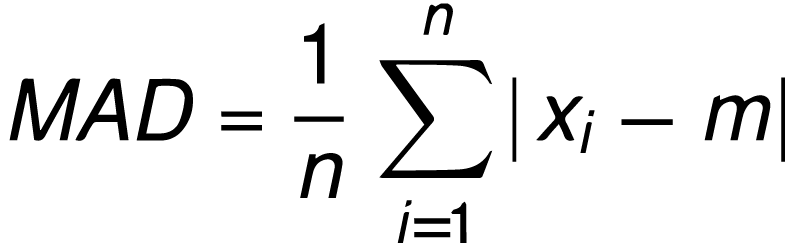Uh Oh! It seems you’re using an Ad blocker!

Since we’ve struggled a lot to makes online calculations for you, we are appealing to you to grant us by disabling the Ad blocker for this domain.# Mean Absolute Deviation Calculator

Central point (m):Enter Central point (m)

Enter the numbers separated by comma ( , )

Table of Content
 1 What is Mean Absolute Deviation (MAD) Formula? 2 MAD Facts: 3 How to Find MAD Manually (Step-by-Step):
Get The Widget!

Add Mean Absolute Deviation Calculator to your website to get the ease of using this calculator directly. Feel hassle-free to account this widget as it is 100% free, simple to use, and you can add it on multiple online platforms.

Available on App

An online mean absolute deviation calculator helps you to find the absolute deviation of the given number around the mean, median or any other number. The mad calculator tells you the measure of dispersion, how much the values in the data set are different from their mean. Keep reading to know about its formula, how to calculate it manual and much more!

## What is Mean Absolute Deviation (MAD) Formula?

The mean deviation or absolute deviation is calculated by the summation of the difference of each value from mean. The formula used by the calculations of MAD is as follows:

$$MAD = Σ|xi – m| / n$$

Where,

xi are the individual values

m is the mean of numbers

n is the total number

• The data distribution can be determined by the standard deviation of the numbers. Both are similar in concept, but the standard deviation gives the deep knowledge of data set with variance etc.
• MAD represents the mean absolute deviation or median absolute deviation. They both are not the same, median is calculated from central point & mean is calculated from the numbers in the mean absolute deviation & median absolute deviation respectively.

## How to Find MAD Manually (Step-by-Step):

The formula for the calculation is discussed earlier, here we have an example:

Example:

Calculate the mean absolute deviation for the data set: 4, 16, 8, 9, 14, 5?

Solution:

Step 1:

The formula is:

$$MAD = Σ|xi – m| / n$$

Here,

• x1 = 4
• x2 = 16
• x3 = 8
• x4 = 9
• x5 = 14
• x6 = 5

Step 2:

Find the mean:

$$m = 4 + 16 + 8 + 9 + 14 + 5 / 6$$

$$m = 56 / 6$$

$$m = 9.33$$

Step 3:

Find deviation from the mean:

$$4 – 9.33 = -5.33$$

$$16 – 9.33 = 6.67$$

$$8 – 9.33 = -1.33$$

$$9 – 9.33 = -0.33$$

$$14 – 9.33 = 4.67$$

$$5 – 9.33 = -4.33$$

Step 4:

Determine the mode of each value:

$$|-5.33| = 5.33$$

$$|6.67| = 6.67$$

$$|-1.33|= 1.33$$

$$|-0.33| = 0.33$$

$$|4.67| = 4.67$$

$$|-4.33| = 4.33$$

Step 5:

Determine the mean of absolute values:

$$m = 5.33 + 6.67 + 1.33 + 0.33 + 4.67 + 4.33 / 6$$

$$m = 22.66 / 6$$

$$m = 3.8$$

Also, our mean deviation calculator uses this formula for the calculation of the MAD. For ease, you can also try our online mean mode median calculator that helps you to calculate the mean, mode & median of your given data set.

The calculation becomes very easy with this online Mean Absolute Deviation Calculator. Just stick to the following points:

Inputs:

• First of all, choose from the dropdown menu around which the data is dispersed.
• Very next, enter the numbers in the designated field.
• Lastly, hit the calculate button.

Note:

If you select the ‘other’ from the dropdown menu, then enter the value around which the data is distributed.

Outputs:

Once you enter in all the fields, the Mad calculator shows:

• Mean Absolute Deviation of data set.
• Step-by-Step Calculation.

## End-Note:

Remember that, MAD assists to get a sense how the values are spread out in a data set. It is helpful for the data analysis in the Probability & Statistics. Quit worrying about its calculations; simply use an online mean deviation calculator that helps you to do the calculation easy & accurately.

## References:

From the authorized source of Wikipedia: Definition & formula of MAD

From the site of Khanacademy : How to do the calculations